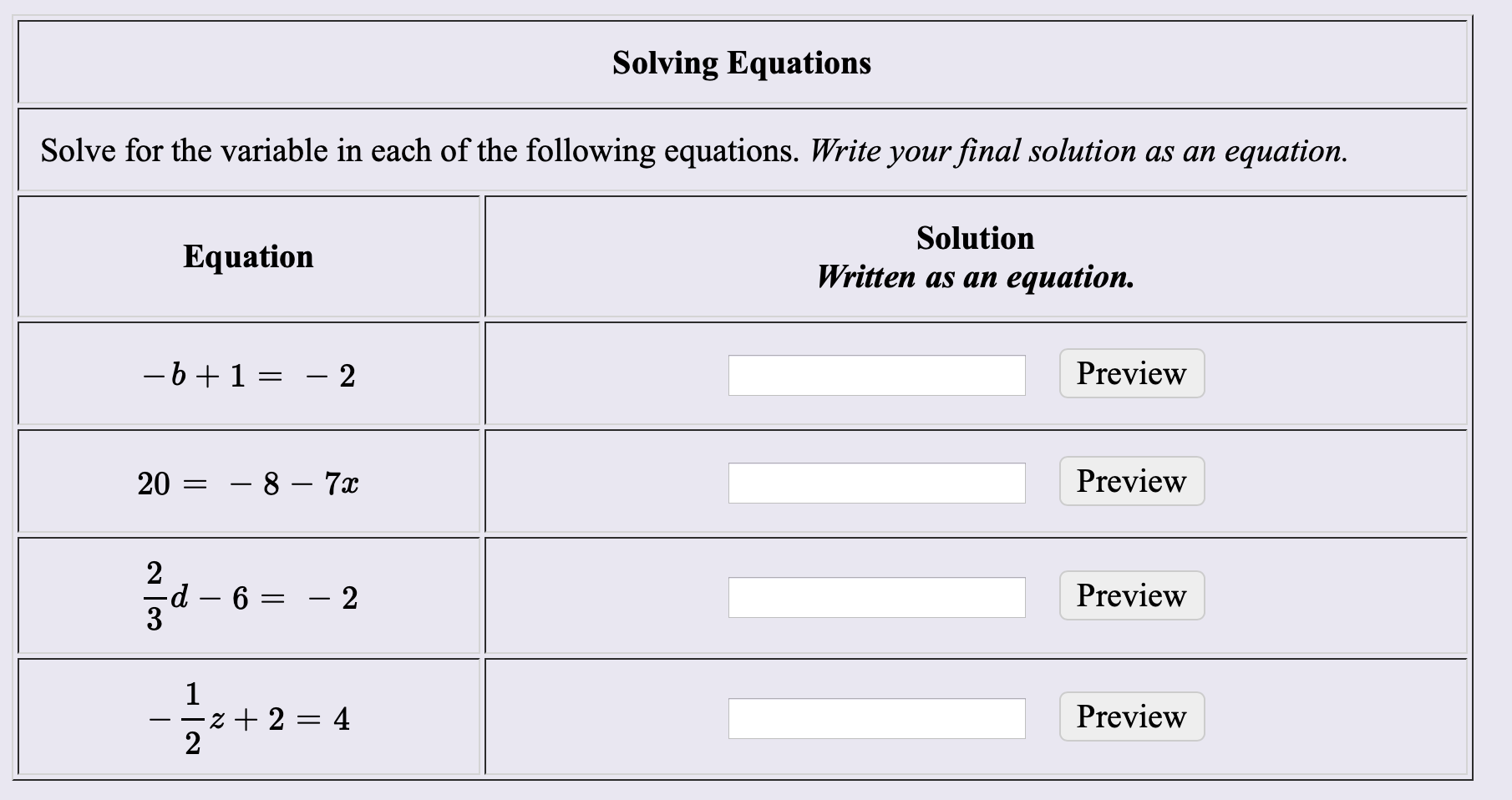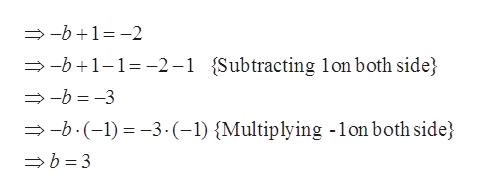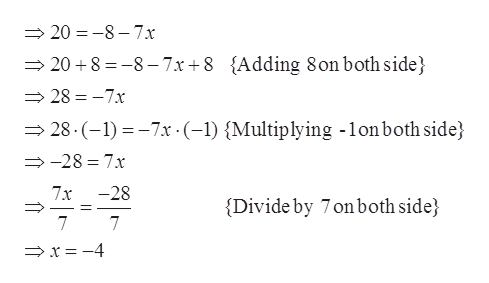Solving EquationsSolve for the variable in each of the following equations. Write your final solution as an equationSolutionEquationWritten as anequation.-b 1 =Preview2Preview- 8 - 7x202d- 2Preview61z 2 = 42Preview

Questionhelp_outlineImage TranscriptioncloseSolving Equations Solve for the variable in each of the following equations. Write your final solution as an equation Solution Equation Written as an equation. -b 1 = Preview 2 Preview - 8 - 7x 20 2 d - 2 Preview 6 1 z 2 = 4 2 Preview fullscreen
Step 1

Since we only answer up to 3 sub-parts, we’ll answer the first 3. Please resubmit the question and specify the other subparts (up to 3) you’d like answered

Step 2

Equation defined as the values of two mathematical expressions are equal.

Part (a):help_outlineImage Transcriptionclose-b1-2 -b1-1-2- Subtracting lonboth side -b-3 -b. (-1)3.(-1) {Multiplying -1on both side b 3 fullscreen
Step 3

Part (b)...help_outlineImage Transcriptionclose20 -8-7x {Adding 8onboth side} 20 8 -8-7x+8 28-7x 28.(-1)7x (-1) Multiplying -lonboth side} -28 7x -28 7x Divide by 7 on both side 7 7 x-4 fullscreen

Want to see the full answer?

See Solution

Want to see this answer and more?

Our solutions are written by experts, many with advanced degrees, and available 24/7

See Solution
Tagged in

Algebra